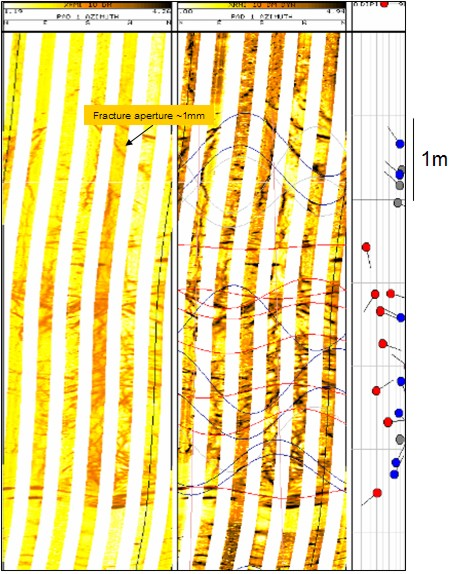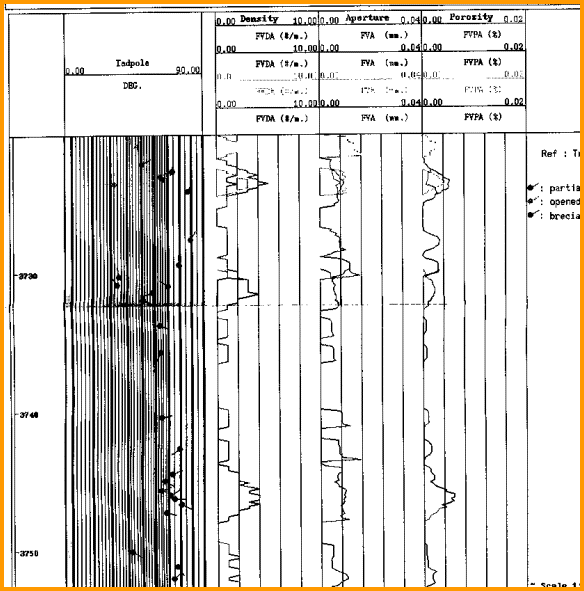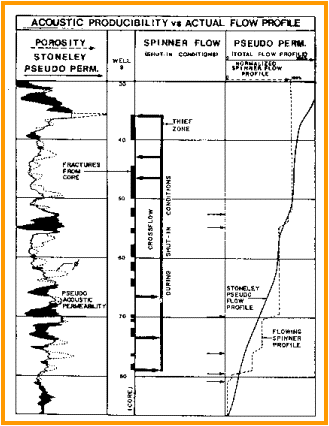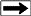Fracture Porosity and Permeability From Fracture Aperture
Resistivity image logs are widely used to assess fracture aperture. Unfortunately, the image tends to exaggerate fracture aperture, especially for very small fractures. The fracture noted on the image at the right looks to be about 1 mm aperture (black streak on the image). This is about the minimum size that  a fracture can appear on a log because of the pixel density of the image, electrode spacing on the tool, and erosion of the wellbore adjacent to the fracture. The fracture frequency may also be exaggerated if the dip correlation processing picks the same fracture at different depths. If fracture dios are hand-picked, fracture frequency will be more accurate.

Fracture aperture exaggeration on acoustic image logs is even more severe and these logs probably should not be used for aperture estimation.

These visual difficulties can be overcome with a post-processing technique that uses a resistivity inversion model and the mud filtrate resistivity to calculate aperture, independent of any visual artifacts. The algorithm is based on the concept that higher electrical conductivity means a larger open fracture. The fracture aperture and fracture frequency can be combined to obtain fracture porosity and fracture permeability.
1. PHIf = 0.001 * Wf * Df * KF1

The fracture permeability equations are attributed to Dr Zoltan Barlai:
2: Kfrac = 833 * 10^11 * PHIfrac^3 / (Df^2 * KF1^2)
3: Kfrac = 833 * 10^5 * PHIfrac * Wf^2
4: Kfrac = 833 * 10^2 * Wf^3 * Df * KF1Where:
KF1 = number of main fracture directions
= 1 for sub-horizontal or sub-vertical
= 2 for orthogonal sub-vertical
= 3 for chaotic or brecciated
PHIfrac = fracture porosity (fractional)
Df = fracture frequency (fractures per meter)
Wf = fracture aperture (millimeters)
Kfrac = fracture permeability (millidarcies)

Note: Equations 2, 3, and 4 give identical results.

Example 1:
Df = 1 fracture per meter
Wf = 1.0 millimeters
PHIfrac = 0.001 * 1 * 1 = 0.001 fractional (0.1%)
Kfrac = 833 * 100 * 1^3 * 1 * 1 = 83300 millidarcies

Example 2:
Df = 10 fractures per meter
Wf = 0.1 millimeters
PHIfrac = 0.001 * 10 * 0.1 = 0.001 fractional (0.1%)
Kfrac = 833 * 100 * 0.1^3 * 10 * 1 = 833 millidarcies

These examples represent well fractured reservoirs. You can see that the volume of hydrocarbon is very small but the permeability is very high.

If you believe that the phrase “fracture porosity” is a literal definition, then this porosity will usually be pretty small - in the order of 0.0001 to 0.01 fractional porosity (0.01 to 1.0%). If you believe that the phrase includes vuggy and solution porosity related to the presence of fractures, then the value could be much higher. The important thing is to recognize that there are two definitions for “fracture porosity”.

An example of a fracture aperture log from a program called Frac-View is shown below. The permeability calculation was not available in this program.Fracture frequency, aperture, and porosity log derived from a resistivity image log.Calculating Permeability From Stoneley AttenuationWhile propagating along the borehole wall, the Stoneley wave is able to exchange energy with the formation fluid in a process called acoustic flow. This communication between the borehole and formation is proportional to the mobility of the fluids, which in turn is proportional to permeability and fluid viscosity. Increases in communication decrease Stoneley amplitude, because energy is used up when acoustic flow is initiated. This is equivalent to increased Stoneley attenuation, which therefore can be calibrated to predict formation permeability.

Permeability from Stoneley wave attenuationThe attenuation data can be represented as pseudo-permeability and presented on a qualitative scale: low attenuation corresponding to low permeability and high attenuation to high permeability. To facilitate comparisons of pseudo-permeability to actual formation producibility, the attenuation data can be integrated to provide a potential flow profile for comparison to an actual spinner flowmeter log. .

This curve could be correlated to core permeability to obtain a calibrated permeability curve. Correlation is usually as good as porosity and saturation correlations, which have been used for many years, and often much better than these in fractured and vuggy zones.

Page Views ---- Since 01 Jan 2015
Copyright 2023 by Accessible Petrophysics Ltd.
CPH Logo, "CPH", "CPH Gold Member", "CPH Platinum Member", "Crain's Rules", "Meta/Log", "Computer-Ready-Math", "Petro/Fusion Scripts" are Trademarks of the Author